# Equations of Motion

This section gives the equations for a trebuchet. I would suggest working through this Example Problem to understand the basics of how the equations work before trying work through this section.

The equations of motion for the trebuchet are a set of second order differential equations. The numerical methods used in the simulation only work with first order differential equations, so the derivatives of the motion variables were also renamed as separate variables which allowed the equations of motion to be rewritten as a set of first order differential equations. Those first order differential equations are presented in this section. There are a different set of equations for each stage of the trebuchet as described in the Simulation Overview.

The equations of motion for the trebuchet were derived using a program called Autolev. See the Autolev section to see the code that was actually used to develop the equations of motion.

## Stage 1:

In stage 1 the motion variables are Aq, Wq, and Sq, and the constant lengths are LAl, LAs, LAcg, LW, LS, and h.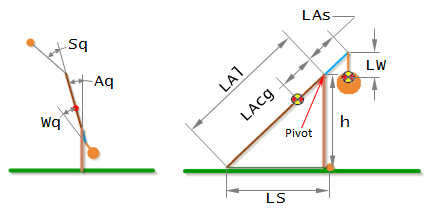The time derivative of a variable is denoted by appending it with a '. For example Aq' means dAq/dt, and Sq'' means d²Sq/dt².

Additional constants not shown above are:

Grav = Gravitational Acceleration

mA = Mass of Arm

mW = Mass of Weight

mP = Mass of Projectile

IA3 = Inertia of Arm

IW3 = Inertia of Weight

The equations of motion for stage 1 are:

```(-mP*LAl^2*(-1+2*SIN(Aq)*COS(Sq)/SIN(Aq+Sq)) + IA3 + IW3 + mA*LAcg^2 + mP*LAl^2*SIN(Aq)^2/SIN(Aq+Sq)^2 + mW*(LAs^2+LW^2+2*LAs*LW*COS(Wq)))*````Aq''` `+ (IW3 + LW*mW*(LW+LAs*COS(Wq)))*``Wq''` ```= Grav*LAcg*mA*SIN(Aq) + LAl*LS*mP*(SIN(Sq)*(Aq'+Sq')^2+COS(Sq)*(COS(Aq+Sq)*Sq'*(Sq'+2*Aq')/SIN(Aq+Sq)+(COS(Aq+Sq)/SIN(Aq+Sq)+LAl*COS(Aq)/(LS*SIN(Aq+Sq)))*Aq'^2)) + LAl*mP*SIN(Aq)*(LAl*SIN(Sq)*Aq'^2-LS*(COS(Aq+Sq)*Sq'*(Sq'+2*Aq')/SIN(Aq+Sq)+(COS(Aq+Sq)/SIN(Aq+Sq)+LAl*COS(Aq)/(LS*SIN(Aq+Sq)))*Aq'^2))/SIN(Aq+Sq) - Grav*mW*(LAs*SIN(Aq)+LW*SIN(Aq+Wq)) - LAs*LW*mW*SIN(Wq)*(Aq'^2-(Aq'+Wq')^2)```

`(IW3 + LW*mW*(LW+LAs*COS(Wq)))*``Aq''` `+ (IW3 + mW*LW^2)*``Wq''` `= -LW*mW*(Grav*SIN(Aq+Wq)+LAs*SIN(Wq)*Aq'^2)`

Note that in stage 1, when the ball is on the ground, the trebuchet only has two degrees of freedom, which is why there are only two equations of motion. However there were three variables to begin with. This works because Sq’ becomes a dependent variable defined by the expression,

`Sq'``= -(LAl*SIN(Aq)+LS*SIN(Aq+Sq))*Aq'/(LS*SIN(Aq+Sq))`

and Sq’’ becomes,

`Sq''````= -COS(Aq+Sq)*Sq'*(Sq'+2*Aq')/SIN(Aq+Sq) - (COS(Aq+Sq)/SIN(Aq+Sq)+LAl*COS(Aq)/(LS*SIN(Aq+Sq)))*Aq'^2 - (LAl*SIN(Aq)+LS*SIN(Aq+Sq))*Aq''/(LS*SIN(Aq+Sq))```

As is discussed in the Example Problem, complex differential equations like this are practically impossible to solve analytically. As a result, numerical methods must be used to approximate an answer. These methods require that the equations of motion to be reduced to first order differential equations. In order to accomplish this, we will define three new variables Aw, Ww, and Sw which are the the time derivatives of Aq, Wq, and Sq.

`Aw = Aq'`

`Ww = Wq'`

`Sw = Sq'`

Substituting Aw, Ww, and Sw in to the equations of motion gives,

```(-mP*LAl^2*(-1+2*SIN(Aq)*COS(Sq)/SIN(Aq+Sq)) + IA3 + IW3 + mA*LAcg^2 + mP*LAl^2*SIN(Aq)^2/SIN(Aq+Sq)^2 + mW*(LAs^2+LW^2+2*LAs*LW*COS(Wq)))*````Aw'` `+ (IW3 + LW*mW*(LW+LAs*COS(Wq)))*``Ww'` ```= Grav*LAcg*mA*SIN(Aq) + LAl*LS*mP*(SIN(Sq)*(Aw+Sw)^2+COS(Sq)*(COS(Aq+Sq)*Sw*(Sw+2*Aw)/SIN(Aq+Sq)+(COS(Aq+Sq)/SIN(Aq+Sq)+LAl*COS(Aq)/(LS*SIN(Aq+Sq)))*Aw^2)) + LAl*mP*SIN(Aq)*(LAl*SIN(Sq)*Aw^2-LS*(COS(Aq+Sq)*Sw*(Sw+2*Aw)/SIN(Aq+Sq)+(COS(Aq+Sq)/SIN(Aq+Sq)+LAl*COS(Aq)/(LS*SIN(Aq+Sq)))*Aw^2))/SIN(Aq+Sq) - Grav*mW*(LAs*SIN(Aq)+LW*SIN(Aq+Wq)) - LAs*LW*mW*SIN(Wq)*(Aw^2-(Aw+Ww)^2)```

`(IW3 + LW*mW*(LW+LAs*COS(Wq)))*``Aw'` `+ (IW3 + mW*LW^2)*``Ww'` `= -LW*mW*(Grav*SIN(Aq+Wq)+LAs*SIN(Wq)*Aw^2)`

which are now first order differntial equations. As discussed in the Example Problem, we need to get the equations into the form,

`y'(t) = f(t, y(t))`

Separating Aw’ and Ww’ into separate expressions will require a little linear algebra. So, first we will write the equations of motion in matrix form.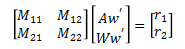where,

`M11````= -mP*LAl^2*(-1+2*SIN(Aq)*COS(Sq)/SIN(Aq+Sq)) + IA3 + IW3 + mA*LAcg^2 + mP*LAl^2*SIN(Aq)^2/SIN(Aq+Sq)^2 + mW*(LAs^2+LW^2+2*LAs*LW*COS(Wq))```

`M12``= IW3 + LW*mW*(LW+LAs*COS(Wq))`

`M21``= IW3 + LW*mW*(LW+LAs*COS(Wq))`

`M22``= IW3 + mW*LW^2`

`r1````= Grav*LAcg*mA*SIN(Aq) + LAl*LS*mP*(SIN(Sq)*(Aw+Sw)^2+COS(Sq)*(COS(Aq+Sq)*Sw*(Sw+2*Aw)/SIN(Aq+Sq)+(COS(Aq+Sq)/SIN(Aq+Sq)+LAl*COS(Aq)/(LS*SIN(Aq+Sq)))*Aw^2)) + LAl*mP*SIN(Aq)*(LAl*SIN(Sq)*Aw^2-LS*(COS(Aq+Sq)*Sw*(Sw+2*Aw)/SIN(Aq+Sq)+(COS(Aq+Sq)/SIN(Aq+Sq)+LAl*COS(Aq)/(LS*SIN(Aq+Sq)))*Aw^2))/SIN(Aq+Sq) - Grav*mW*(LAs*SIN(Aq)+LW*SIN(Aq+Wq)) - LAs*LW*mW*SIN(Wq)*(Aw^2-(Aw+Ww)^2)```

`r2``= -LW*mW*(Grav*SIN(Aq+Wq)+LAs*SIN(Wq)*Aw^2)`

We can solve for Aw' and Ww' by multiplying both sides of the equation by the inverse of matrix M.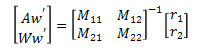which give the result,

`Aw'``= (r1*M22-r2*M12)/(M11*M22-M12*M21)`

`Ww'``= -(r1*M21-r2*M11)/(M11*M22-M12*M21)`

In summary, the equations that are used by the simulation for stage 1 are,

`Aq'``= Aw`

`Wq'``= Ww`

`Sq'``= Sw`

`Aw'``= (r1*M22-r2*M12)/(M11*M22-M12*M21)`

`Ww'``= -(r1*M21-r2*M11)/(M11*M22-M12*M21)`

`Sw'````= -COS(Aq+Sq)*Sq'*(Sq'+2*Aq')/SIN(Aq+Sq) - (COS(Aq+Sq)/SIN(Aq+Sq)+LAl*COS(Aq)/(LS*SIN(Aq+Sq)))*Aq'^2 - (LAl*SIN(Aq)+LS*SIN(Aq+Sq))*Aq''/(LS*SIN(Aq+Sq))```

As described in the Example Problem, these first order differential equations are solved using the numerical methods described in the Runge-Kutta section, and the results are values for the angles (Aq, Wq, Sq) and their derivatives (Aw, Ww, Sw) over time. These angles can be converted into X and Y coordinates using the expressions found in the Angles to XY Coordinates section.

## Stage 2:

In stage 2 the parameters are the same as in stage 1. The equations of motion for stage 2 are:

```(IA3 + IW3 + mA*LAcg^2 + mP*(LAl^2+LS^2+2*LAl*LS*COS(Sq)) + mW*(LAs^2+LW^2+2*LAs*LW*COS(Wq)))*````Aq''` `+ (IW3 + LW*mW*(LW+LAs*COS(Wq)))*``Wq''` `+ (LS*mP*(LS+LAl*COS(Sq)))*``Sq''` ```= Grav*LAcg*mA*SIN(Aq) + Grav*mP*(LAl*SIN(Aq)+LS*SIN(Aq+Sq)) - Grav*mW*(LAs*SIN(Aq)+LW*SIN(Aq+Wq)) - LAl*LS*mP*SIN(Sq)*(Aq'^2-(Aq'+Sq')^2) - LAs*LW*mW*SIN(Wq)*(Aq'^2-(Aq'+Wq')^2)```

`(IW3 + LW*mW*(LW+LAs*COS(Wq)))*``Aq''` `+ (IW3 + mW*LW^2)*``Wq''` `= -LW*mW*(Grav*SIN(Aq+Wq)+LAs*SIN(Wq)*Aq'^2)`

`(LS*mP*(LS+LAl*COS(Sq)))*``Aq''` `+ (mP*LS^2)*``Sq''` `= LS*mP*(Grav*SIN(Aq+Sq)-LAl*SIN(Sq)*Aq'^2)`

Note that in stage 2, there are three degrees of freedom, so there are now three equations of motion. As in stage 1 we will turn the equations of motion onto first order differential equations by using the time derivatives of Aq, Wq, and Sq, which we will call Aw, Ww, and Sw, and substituting them into the equations of motion.

```(IA3 + IW3 + mA*LAcg^2 + mP*(LAl^2+LS^2+2*LAl*LS*COS(Sq)) + mW*(LAs^2+LW^2+2*LAs*LW*COS(Wq)))*````Aw'` `+ (IW3 + LW*mW*(LW+LAs*COS(Wq)))*``Ww'` `+ (LS*mP*(LS+LAl*COS(Sq)))*``Sw'` ```= Grav*LAcg*mA*SIN(Aq) + Grav*mP*(LAl*SIN(Aq)+LS*SIN(Aq+Sq)) - Grav*mW*(LAs*SIN(Aq)+LW*SIN(Aq+Wq)) - LAl*LS*mP*SIN(Sq)*(Aw^2-(Aw+Sw)^2) - LAs*LW*mW*SIN(Wq)*(Aw^2-(Aw+Ww)^2)```

`(IW3 + LW*mW*(LW+LAs*COS(Wq)))*``Aw'` `+ (IW3 + mW*LW^2)*``Ww'` `= -LW*mW*(Grav*SIN(Aq+Wq)+LAs*SIN(Wq)*Aw^2)`

`(LS*mP*(LS+LAl*COS(Sq)))*``Aw'` `+ (mP*LS^2)*``Sw'` `= LS*mP*(Grav*SIN(Aq+Sq)-LAl*SIN(Sq)*Aw^2)`

In order to separate Aw’, Ww’, and Sw’ into separate expressions we will first put the equations of motion into matrix form.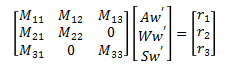where,

`M11````= IA3 + IW3 + mA*LAcg^2 + mP*(LAl^2+LS^2+2*LAl*LS*COS(Sq)) + mW*(LAs^2+LW^2+2*LAs*LW*COS(Wq))```

`M12``= IW3 + LW*mW*(LW+LAs*COS(Wq))`

`M13``= LS*mP*(LS+LAl*COS(Sq))`

`M21``= IW3 + LW*mW*(LW+LAs*COS(Wq))`

`M22``= IW3 + mW*LW^2`

`M31``= LS*mP*(LS+LAl*COS(Sq))`

`M33``= mP*LS^2`

`r1````= Grav*LAcg*mA*SIN(Aq) + Grav*mP*(LAl*SIN(Aq)+LS*SIN(Aq+Sq)) - Grav*mW*(LAs*SIN(Aq)+LW*SIN(Aq+Wq)) - LAl*LS*mP*SIN(Sq)*(Aw^2-(Aw+Sw)^2) - LAs*LW*mW*SIN(Wq)*(Aw^2-(Aw+Ww)^2)```

`r2``= -LW*mW*(Grav*SIN(Aq+Wq)+LAs*SIN(Wq)*Aw^2)`

`r3``= LS*mP*(Grav*SIN(Aq+Sq)-LAl*SIN(Sq)*Aw^2)`

Now, we can solve for Aw', Ww', and Sw’ by multiplying both sides of the equation by the inverse of matrix M.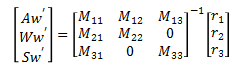which gives the result used in the simulation,

`Aq'``= Aw`

`Wq'``= Ww`

`Sq'``= Sw`

`Aw'``= -(r1*M22*M33-r2*M12*M33-r3*M13*M22)/(M13*M22*M31-M33*(M11*M22-M12*M21))`

`Ww'``= (r1*M21*M33-r2*(M11*M33-M13*M31)-r3*M13*M21)/(M13*M22*M31-M33*(M11*M22-M12*M21))`

`Sw'``= (r1*M22*M31-r2*M12*M31-r3*(M11*M22-M12*M21))/(M13*M22*M31-M33*(M11*M22-M12*M21))`

As described in the Example Problem, these first order differential equations are solved using the numerical methods described in the Runge-Kutta section, and the results are values for the angles (Aq, Wq, Sq) and their derivatives (Aw, Ww, Sw) over time. These angles can be converted into X and Y coordinates using the expressions found in the Angles to XY Coordinates section.

## Stage 3:

Stage 3 has a different set of parameters than stage 1 or 2. The motion variables are Px and Py. Note that in the simulation everything is measured relative to the pivot point.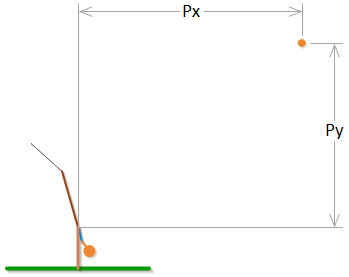The constant parameters in stage 3 are:

Grav = Gravitational Acceleration ρ = Density of Air

WS = Wind Speed

Aeff = Effective Area of the Projectile (= π*(Projectile Diameter/2)^2)

mP = Mass of Projectile

Cd = Drag Coefficient of Projectile

The drag coefficient is not actually a constant. It is related to the Reynolds number which is dependent on the velocity of the projectile relative to the air. For more information on how the drag coefficient is derived, see the Projectile Drag section.

The equations of motion for stage 3 are:

`Px''``= -(ρ*Cd*Aeff*(Px'-WS)*sqrt(Py'^2+(WS-Px')^2))/(2*mP)`

`Py''``= -Grav - (ρ*Cd*Aeff*Py'*sqrt(Py'^2+(WS-Px')^2))/(2*mP)`

In order to make the equations of motion into first order differential equations, we will define to new variables Pvx and Pvy which we will make equal to the time derivatives of Px and Py.

`Pvx = Px'`

`Pvy = Py'`

Substituting these into the equations of motion gives the expressions used in the simulation.

`Px'``= Pvx`

`Py'``= Pvy`

`Pvx'``= -(ρ*Cd*Aeff*(Pvx-WS)*sqrt(Pvy^2+(WS-Pvx)^2))/(2*mP)`

`Pvy'``= -Grav - (ρ*Cd*Aeff*Pvy*sqrt(Pvy^2+(WS-Pvx)^2))/(2*mP)`

As described in the Example Problem, these first order differential equations are solved using the numerical methods described in the Runge-Kutta section, and the results are values for the variables (Px, Py) and their derivatives (Pvx, Pvy) over time.

 Units English (feet)English (inches)Metric Length of Short Arm ft Length of Long Arm ft Length of Sling ft Length of Weight ft Height of Pivot ft Uniform arm Mass of Arm lb Inertia of Arm lb·ft² Pivot to Arm CG ft Mass of Weight lb Inertia of Weight lb·ft² Projectile BaseballGolf BallPumpkinCowCustom Mass of Projectile lb Projectile Diameter ft Wind Speed ft/s Release Angle ° Play speed: 1x Link to save this trebuchet

## VirtualTrebuchet 2.0

VirtualTrebuchet is a web based trebuchet simulator that will allow you to quickly evaluate different trebuchet configurations.

1. To begin, enter the parameters of your trebuchet in the input boxes.
2. Next, press the "Simulate" button located under the inputs.
3. Finally, watch your trebuchet go.